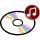Dr. S. R. Lasker Library Online Catalogue

Normal view

# Physics of optoelectronics / Michael A. Parker.

Material type:TextLanguage: English Publication details: 2005. Description: xvi, 742 p. : ill. ; 26 cmISBN: 0824753852; 9780824753856Other title: OptoelectronicsSubject(s): Optoelectronics -- MaterialsDDC classification: 621.381045 LOC classification: TA1750 | .P37 2005Online resources: Publisher description | WorldCat details | E-book Fulltext
Contents:
TOC Ch. 1. Introduction to semiconductor lasers -- Ch. 2. Introduction to laser dynamics -- Ch. 3. Classical electromagnetics and lasers -- Ch. 4. Mathematical foundations -- Ch. 5. Fundamentals of dynamics -- Ch. 6. Light -- Ch. 7. Matter-light interaction -- Ch. 8. Semiconductor emitters and detectors -- App. 1. Review of integrating factors -- App. 2. Rate and continuity equations -- App. 3. The group velocity -- App. 4. Review of probability theory and statistics -- App. 5. The Dirac delta function -- App. 6. Coordinate representations of the Schrodinger wave equation -- App. 7. Integrals with two time scales -- App. 8. The dipole approximation -- App. 9. The density operator and the Boltzmann distribution.
Summary: Summary: Focuses on the properties of optical fields and their interaction with matter. This work offers an introduction to lasers, LEDs, and the rate equations, and then describes the emission and detection processes. It summarizes and reviews the mathematical background of the quantum theory embodied in the Hilbert space.
Tags from this library: No tags from this library for this title.
Star ratingsAverage rating: 0.0 (0 votes)
Holdings
Item type Current library Collection Call number Copy number Status Date due Barcode Item holdsE-Book
E-book
Non-fiction 621.381045 PAP 2005 (Browse shelf(Opens below)) Not for loanText
Reserve Section
Non-fiction 621.381045 PAP 2005 (Browse shelf(Opens below)) C-1 Not For Loan 21168CDs & DVDs
Audio Visual
Non-fiction 621.381045 PAP 2005 (Browse shelf(Opens below)) C-1 Available CD-1297Text
Circulation Section
Non-fiction 621.381045 PAP 2005 (Browse shelf(Opens below)) C-2 Available 21169CDs & DVDs
Audio Visual
Non-fiction 621.381045 PAP 2005 (Browse shelf(Opens below)) C-2 Available CD-1298
Total holds: 0

Includes bibliographical references and index.

TOC Ch. 1. Introduction to semiconductor lasers --
Ch. 2. Introduction to laser dynamics --
Ch. 3. Classical electromagnetics and lasers --
Ch. 4. Mathematical foundations --
Ch. 5. Fundamentals of dynamics --
Ch. 6. Light --
Ch. 7. Matter-light interaction --
Ch. 8. Semiconductor emitters and detectors --
App. 1. Review of integrating factors --
App. 2. Rate and continuity equations --
App. 3. The group velocity --
App. 4. Review of probability theory and statistics --
App. 5. The Dirac delta function --
App. 6. Coordinate representations of the Schrodinger wave equation --
App. 7. Integrals with two time scales --
App. 8. The dipole approximation --
App. 9. The density operator and the Boltzmann distribution.

Summary:
Focuses on the properties of optical fields and their interaction with matter. This work offers an introduction to lasers, LEDs, and the rate equations, and then describes the emission and detection processes. It summarizes and reviews the mathematical background of the quantum theory embodied in the Hilbert space.

EEE

Tahur Ahmed

There are no comments on this title.